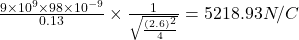Question

A thin plastic rod of length 2.6 m is rubbed all over with wool, and acquires a charge of 98 nC, distributed uniformly over its surface. Calculate the magnitude of the electric field due to the rod at a location 13 cm from the midpoint of the rod. Do the calculation two ways, first using the exact formula for a rod of any length, and second using the approximate formula for a long rod.

1.Thunguyet

By exact formula

5076.59N/C

And by approximation formula

5218.93N/C

Explanation:

We are given that

Length of rod,L=2.6 m

Charge,q=98nC=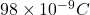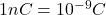a=13 cm=0.13 m

1 m=100 cm

By exact formula

The magnitude of  the electric field due to the rod at a location 13 cm from the midpoint of the rod=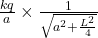Where k=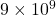Using the formula

The magnitude of  the electric field due to the rod at a location 13 cm from the midpoint of the rod=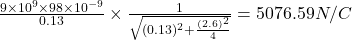In approximation formula

a<<L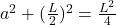Therefore,the magnitude of  the electric field due to the rod at a location 13 cm from the midpoint of the rod=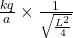The magnitude of  the electric field due to the rod at a location 13 cm from the midpoint of the rod=